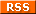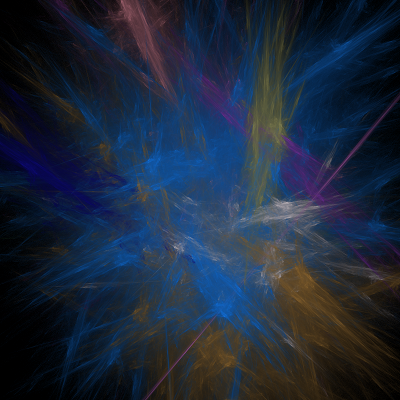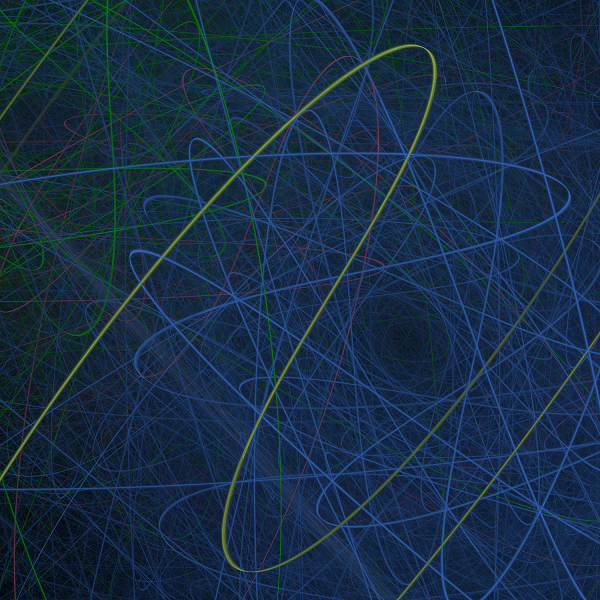# Computer generated artI have a passion for recreational mathematical puzzles. Especially combinatorical puzzles or puzzles involving square numbers. My approach is normally some mathematical research combined with brute force CPU power. Often I am not able to solve the puzzle, but sometimes I am. However even when I fail to find a solution the information gained can be interesting since it can reveal the minimum size of a solution or patterns in the numbers . This adventure is a little unusual since it is not about squares or combinatorics at all.

## Abstract:

### Can you make a computer program that can create 'art'? That was my challenge. My own artistic skills are pathetic and if I ever wanted to create something beautiful, I realized I had to make a program that did it. I was shocked by the stunning beauty of the pictures I saw from the Electric Sheep Project and decided to do something similar. The technique used is called Fractal Flames. Fractal flames is totally different from the 'old school' fractals like the Mandelbrot and have nothing in common except the name 'Fractal'. The image to the right was one of the first fractals my program generated after I had implemented 'logarithmic density coloring' and this was only using the most simple transformations - the linear transformations. It was a good beginning that motivated me to continue working on the project. Splashing some colors together may not be art, but the important thing to notice is that the picture seems to have depth due to the logarithmic density coloring. It actually looks a little like it was painted with a real brush.### Fractal flames explained:

Fractal flames are a completely different breed compared to the 'classic' fractals. They are also newer, fractal flames was 'invented' in 1992 while the classic fractals was 'discovered' in 1979. Unlike classic fractals that basically only had one way to construct them, when creating fractal flames you have a lot more freedom and there is no exact algorithm you have to follow. You are free to put in lot of homemade stuff which will make your fractals unique and give them a personal touch. I will not explain fractal flames in details since it is a longer story and is explained in PDF explaining the Fractal Flames algorithm. I only used this PDF as source of information when creating the program. Later I found a more Easy Explanation of Fractal Flames which will suffice to give an overview of the whole thing.

The fundamental building blocks when creating fractal flames are functions that maps the plane into itself (ℝ2 into ℝ2). These functions are called 'variations' in fractal flames language. One of the variations giving the best results for me is:

Horseshoe variation:v(x,y)=(1/r)*( (x-y)(x+y) , 2xy), where r= Sqrt(x2+y2).

The PDF document describes 48 different variations, but you can always just invent new variations yourself. The basic rule is that the functions has to be 'bounded' somewhat so points near (0,0) also are mapped to a pointer near (0,0) etc. However combining a few of these variations for a fractal only gives a very limited set of fractals. So next step is to expand these variations into infinite many variations.

For any variation v(x,y) you can define the funtion f(x,y)=v(ax+by+c,dx+ey+f) where a,b,c,d,e,f ∈ℝ

You are now ready to make fractal flames. The following algorithm is not the simplest, but the simplest version needed to create something dazzling in my opinion. Start by picking a few (lets say 4) of these variations functions f1(x,y), f2(x,y), f3(x,y), f4(x,y) and assign a (random in my program) color to each of them. For each variation you need a data-structure to keep track of how many times each pixel has 'been hit'. (Ie. pixel (111,222) has been hit 51333 times). This is almost like each variation has its own picture, but except of drawing the pixel with the color, you instead keep track of how many times it has been hit. You can also still visualize this as a normal picture for each variation. If the color for that variation is red, a pixel that has been hit many times (relative!) will be very dark red, while those with low hit-count will be light red. And now comes the fun part, the chaos game algorithm.

The chaos game algorithm:

pick (x,y) random in the unit square.
iterate 'enough times' {
pick random variation fi(x,y) from you set of variations, which is 4 in this example
(x,y):=fi(x,y)
increase hit count for pixel (x,y) for variation fi.
}

Draw the fractal by 'superposing' the 4 variations (pixel count in that color) on top of each other. Use color-merging algorithm you think is fair.

You can add a few more steps in the algorithm to introduce some more 'chaos' to you fractals. I also implemented two different symmetry-transformations. Mirror in x-axis, y-axis or both or rotation-symmetry. This is indeed a cheap way to create something our brains like to look at. Humans like symmetry, faces are (almost) symmetric and this is burned into our brains. The y-axis mirroring very often turns some random dots and lines into something more esthetic. But I find this a cheap trick and dont use it much and it never creates the most beautiful fractals. For that you need something fascinating to start with. I also implemented a 'Post Transform' and 'Final Transform' step which are described in one of the PDF's. I also implemented gamma-factor when drawing of the fractals and weighting of the fractal(when picked in the chaos game), but these improvements are very minor. Currently I am now adding another step as well, which is standard image transformations you know from photo-shop (sharpen the picture edges, remove noise, make it look like broken glass etc.) I think you get the picture - you are free to do a lot of stuff when creating fractal flames!

### Algorithm:

The only other algorithm worth mentioning is my weighted-logarithmic algorithm since this drastially improved the fractals that the program generated. Due to the mathematics of the fractal flame algorithm (chaos game) the distribution of the pixels hit will be logarithmic. This means there will be many pixels only hit 10 times, but also many hit 1000 times and also many hit 1000000 times etc. If you do not use logarithmic scale, the small details with few hits will be wiped out when drawing the picture. The final color a pixel is a weighted average of all pixel colors involved and this gives the fractals the 3D look or depth. The fractal will have a wide smooth range of colors and not just the few colors originally assigned to the variations. This draw(rendering) algorithm can take up to 30 seconds if there are many variations involved (and mirror/rotation). But this is not important, since you only need to draw it once after your full fractal generation algorithm is finished.

### Logarithmic coloring algorithm (Java)

 ``` for (int x=0 ; x < width ; x++){ for (int y=0 ; y < height ; y++){ double r=0; double g=0; double b=0; int totalHits = getTotalPixelHitsInAllVariations(drawableVariations,x,y); boolean pixelHit=false; for (int i=0 ; i < drawableVariations.length ; i++){ Variation v = drawableVariations[i]; if (!v.isDraw()) continue; int hits= v.getHits()[x][y]; if ( hits >0 ){ pixelHit=true; double weightFactor=(1d*hits)/(1d*totalHits); r+=v.getColor().getRed()*weightFactor; g+=v.getColor().getGreen()*weightFactor; b+=v.getColor().getBlue()*weightFactor; } } if (pixelHit){ //RGB is now weigthed average totalPixelsHit++; double logFactor=Math.log(totalHits)/Math.log(maxSinglePixelHit); r=Math.min(255,Math.pow(r*logFactor/255,1/config.getGamma())*255); g=Math.min(255,Math.pow(g*logFactor/255,1/config.getGamma())*255); b=Math.min(255,Math.pow(b*logFactor/255,1/config.getGamma())*255); screen.setPixel(x,y,new Color((int) r,(int)g,(int) b).getRGB()); // <- All that work to do this } } } } ```

### My fractal flames style:

I normally use between 2 and 5 variations when creating my flames. But I do not draw all variations when rendering the final picture. This is because very often only a few of the variations(each has its own color) show something pretty. I discard maybe 95% of the fractals generated as likely candidates by my program. And of these 5% there are typically only one or two of the variations involved show something pretty. Very often they just render as noise or some lines all over the picture. So I simply choose not to draw those variations. It is not like I can ignore those variations I do not draw, they still are responsible for how the final flame turns out(in the chaos game). This explains why my flames are so simple, often only having one weird twisted shape, compared to the explosions of colors that the fractals at Electric Sheep Project have. I suspect Electric Sheep have a basis of some linear variations which they include when choosing variations for a fractal. All those linear variations will affect the final image by copying it into many smaller identical images and spread them out into a symmetric pattern. Imagine the tail of a peacock, the single pattern (the eye) is copied to multiple symmetric positions. The eye in itself in not that amazing, but the complete tail indeed is. So you could say my fractals only show the eye part. Maybe I will improve my program by using bases of linear variations as well. I always render the fractals in resolutions from 1500*1500 to 6000*6000 pixels and each fractal takes from 20 minutes up to 8 hours of calculations until I think it is done. All of them would be improved by having a longer calculation fase(chaos game) , but eventually progress will be really slow and converge.

### Results:

Enough talking, lets see some fractal flames!

#### My first fractal flames:

The two fractals below was some of the first fractal my program created. This was before I implemented logarithmic coloring. Actually all variations plotted to the screen just overwriting the color the pixel had before it was hit again. This was a simpler version of the chaos game algorithm that I described. Also, I didn't have a GUI for creating the fractals at that time. Note that all patterns are centered around the origin (0,0), this is because I also did not have a post transformation.#### My first fractals with logarithmic coloring:

Right after implementing post transformation and logarithmic coloring my fractals improved a lot. Finally I was getting something back from the almost 100 hours I had spent on the project! When working on the program, time went very fast, because for every small new thing I implemented you could instantly see it in the flames, so I often worked on it for many hours in a row.#### Latest generation of my fractal flames:

These 4 fractals are created by the latest version of my program and show how utterly different fractal flames can be.#### Fractal flames animations:

You can make movies of fractal flames as well. You just take a series of fractal flame pictures and make them into a movie. Between each picture there is a tiny change to the variations involved and the result is a that the fractal flames seem to come alive moving around smoothly while mutating into something else. The following movie is made out of 400 fractal flames pictures. The total rendering time of the 400 pictures was around 8 hours.

### See more of my fractal flames pictures and animations:

If you want to see more of my fractal flames and videos, I have uploaded them to my Google+ account. I think you need to have a Google+ to be able to see them:
See the full album of my fractal flames
I am still working on this project, but my time is limited. Firstly I will create some more fractals, now that the program is ready for it. And after that I have several improvements in mind. One of the new ideas is making a new coloring algorithm. It is still the logarithmic weight, but instead of giving the same variation different shadings of red depending on the hit rate, I will instead rainbow color it. So just one fractal alone will give some real colorful (psychedelic?) pictures. It would be nice to also improve the AI(algorithm) for when to discard a fractal flame.Right now the program, will discard it if too few pixels are hit' (most common cause) or the opposite that almost every pixel is hit in almost the same color.

Here is a screenshot of the main window of my GUI. Click the picture to see it full-size:### Contact information:

If you have any questions or contributions to the problem you can contact me at thomas.egense@gmail.com